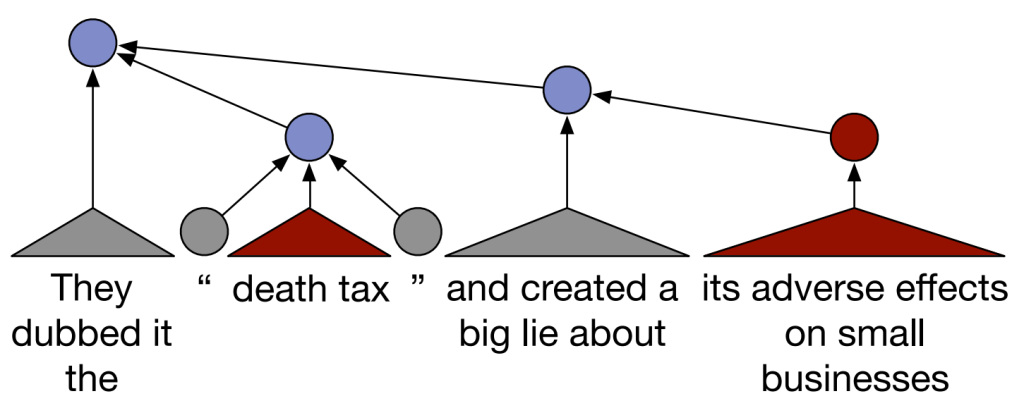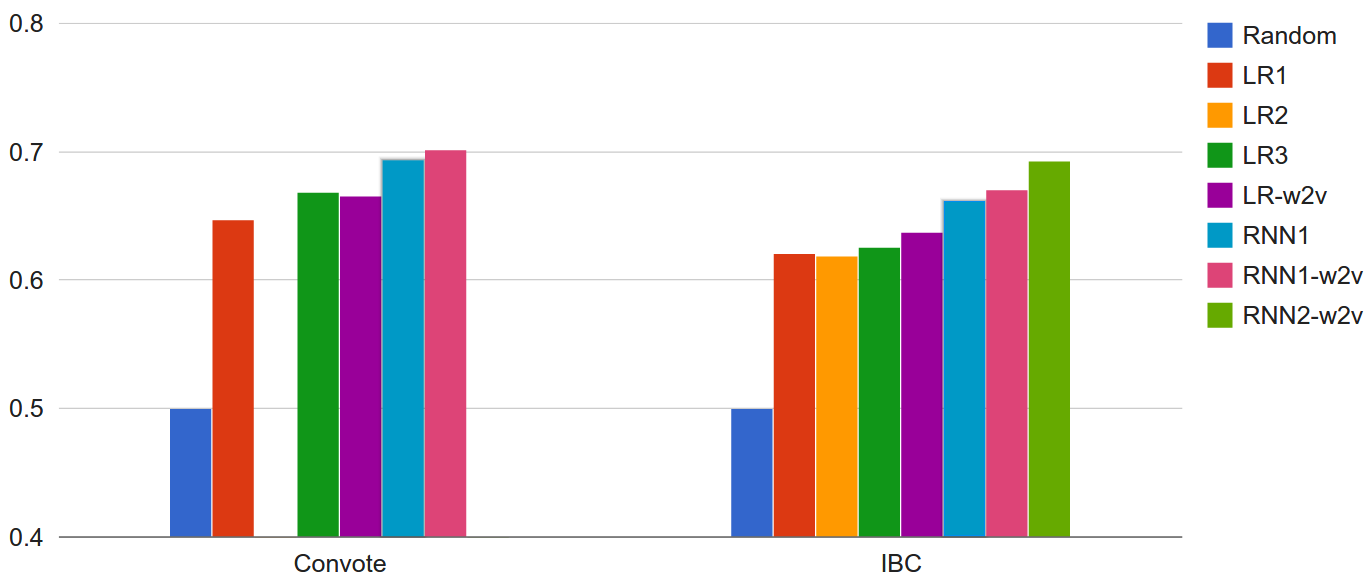# Political ideology detection

Neural networks have a range of interesting applications, and here I will discuss on one them: recursive neural networks and the detection of political ideology. This post is a summary and analysis of a recent publication by Mohit Iyyer, Peter Anns, Jordan Boyd-Graber and Philip Resnik: “Political Ideology Detection Using Recursive Neural Networks“.

Given a sentence, we want the model to detect the political ideology expressed in that sentence. In this research, the authors deal with US politics, so the possible options are liberal (democrats) or conservative (republicans). As a practical application we might consider a system that processes a large amount of news articles or public speeches to detect and measure explicit or hidden political bias of the authors.A traditional approach to this problem is a simple bag-of-words model, where each word is treated as a separate feature, but this ignores any syntactic structure and even word order. As shown below, political ideology can be compositionally complicated – while certain sections of the sentence are locally conservative, the way they are used in context makes the overall sentence liberal.Figure 1: Sample sentence from Iyyer et al. (2014). Blue nodes are liberal, red nodes are conservative, grey nodes are neutral.

## Recursive Neural Network

The recursive neural network in this work is based on Socher et al. (2011), where it was used for sentiment detection. The idea of semantic composition is that the meaning of a sentence or text is composed of its smaller subparts, and recursive neural networks aim to model this by recursively combining vector representations of words and phrases.

We represent each word as a vector of length d. We can then construct a network that takes two word vectors, concatenated into a vector of length 2d, as input and outputs a new vector of length d. We can then stack these networks together and use the output of one network as one of the inputs to another network. This way we can combine multiple words to phrases and phrases to sentences. We don’t need to have a separate network for each of these levels, and can just reuse the same network each time – which is why these are called recursive neural networks.

Mathematically, the composition happens as follows:

$$x_c = f(W_L\times x_a + W_R\times x_b + b)$$

$$x_a$$ and $$x_b$$ are the vectors for the two input words/phrases; $$x_c$$ is the output vector; $$W_L$$ and $$W_R$$ are the weight matrices for the left and right input; $$b$$ is the bias vector. If we think of the input as a concatenation of the vectors, this formula actually becomes the traditional single-layer neural network:

$$x_c = f(W\times x_{ab} + b)$$

An example of this recurrent neural network in action is shown below:During training, an additional softmax layer is added on top, which calculates the probability for both classes (liberal or conservative). The model is then trained to minimize the negative log-probability of the correct class, with L2-regularization.  The error is backpropagated through the whole tree, so the information on the sentence level will be used to modify the individual word vectors, along with all the weights used in the composition.

Also, if I understand the paper correctly, the final prediction from the model at test time is actually generated by averaging vectors for all the nodes in the sentence tree, concatenating this with the root vector, and training a separate logistic regression model over that. Which seems a bit odd, because the recurrent network is quite capable of predicting the class label on the root node, and has been optimised to do so. I wonder what the performance difference would be, if the root-level predictions from the RNN were used directly for evaluation.

## Evaluation

The authors use two datasets for evaluation:

1. The Convote dataset (Thomas et al., 2006) contains transcripts of spoken text from the US Congressional floor debate. The experiments here use 7,816 sentences from the corpus.
2. The Ideological Books Corpus (IBC) (Gross et al., 2013) is a collection of books and articles by authors whose political bias is well-known. Iyyer et al. (2014) extend the dataset by providing sentence-level and partial phrase-level annotation for 4,062 sentences, crowd-sourced through Crowdflower.

Both of these datasets are originally annotated on the level of the author and their political views, but this information can be used to also label each sentence with its most likely political class.

On both datasets, the authors apply heuristic filters to only keep sentences containing explicit bias in either direction, and remove neutral sentences which take up majority of the corpora. However, as this filtering is based on surface forms (essentially bag-of-words or bag-of phrases), it is unknown how this affects the final dataset. It would be interesting to also see results where the test set contains all of the original sentences.

## Results

The authors compare a number of different models.

• Baselines
• Random: The label (conservative/liberal) is chosen randomly.
• LR1: Basic logistic regression using bag-of-words (BoW) features.
• LR2: As previous, but adding phrase annotations as more training data.
• LR3: Logistic regression with BoW and dependency-based features.
• LR-w2v: Logistic regression over averaged word vectors from word2vec.
• RNN Models
• RNN1: The basic RNN model, trained on sentence-level annotations, using random initialization.
• RNN1-w2v: As previous, but initialized with vectors from word2vec.
• RNN2-w2v: The RNN model, trained on sentence-level and phrase-level annotations, initialized with vectors from word2vec.

The results (accuracy) can be seen in the graph.Some conclusions we can draw from the results:

• All the models outperform the random baseline by quite a margin.
• All the recurrent neural network models outperform all the logistic regression models.
• Adding the phrase-level annotations as extra training data for the logistic regression decreases performance (LR2), but adding them to the RNN model improves performance (RNN2-w2v).
• Logistic regression trained on averaged word2vec vectors (LR-w2v) outperforms logistic regression with BoW features (LR1), and even dependency-based features (LR3, on the IBC dataset).
• Initializing the RNN with word2vec vectors gives a little boost to the overall accuracy.

## References

Gross, J., Acree, B., Sim, Y., & Smith, N. A. (2013). Testing the Etch-a-Sketch Hypothesis : Measuring Ideological Signaling via Candidates’ Use of Key Phrases. In APSA 2013.

Iyyer, M., Enns, P., Boyd-Graber, J., & Resnik, P. (2014). Political Ideology Detection Using Recursive Neural Networks. In Proceedings of the 52nd Annual Meeting of the Association for Computational Linguistics (pp. 1113–1122).

Socher, R., Pennington, J., Huang, E. H., Ng, A. Y., & Manning, C. D. (2011). Semi-supervised recursive autoencoders for predicting sentiment distributions. Proceedings of EMNLP. Retrieved from http://dl.acm.org/citation.cfm?id=2145450

Thomas, M., Pang, B., & Lee, L. (2006). Get out the vote: Determining support or opposition from Congressional floor-debate transcripts. In Proceedings of the 2006 Conference on Empirical Methods in Natural Language Processing – EMNLP ’06 (pp. 327–335).

1.Rohan Tondulkar

Hi,
Is the code and dataset available for this paper?

•Marek

Try checking with the authors of the paper

•Deepak

Hi Rohan, any success with dataset and code?